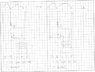# Trouble with calculating 3 Phase Power

• roro36
In summary, In this conversation, the expert summarizer thinks that they should have set the voltage probe to measure power and current instead of power and voltage. They think that if their voltage probe was referenced to earth, their calculations would be accurate.

#### roro36

Hi,

I think I am correct, but just wanted to check. We have some furnaces and I want to work out what power they run at. I have a scope with a current clamp and voltage probe. I have set the scope to read out power and power factor. That is where my problem lies, I think. I should have set it to voltage and pf and read the current off an aditional meter? In any event. It has worked out the power for me. What I want to know, is what is this power value referring to? I attached a drawing that I have used to try and understand it in my head.

Because I have connected the voltage probe to read Line-Line, ie ~400V, the power that it is reading is effectively P=VI*pf.. But it is a 3 phase system. So because I set the voltage probe acoss Line-Line, I need to multiply this Power value by root3 to get the power of the machine. If i had set the voltage probe to read Line-Neutral, I would multiply the power reading by 3? I know I have assume equal currents and that the meter is working out single phase Power I think, the setting just says Power and says Voltage Probe A, Current Probe B.

Please let me know if this correct.

#### Attachments

•Power.jpg
49.8 KB · Views: 498
Without knowing the specifics of you scope/meter I think that you may get an anomalous power factor reading due to the voltage measurement being line to line. That is, (unless the scope/meter somehow knows this and is correcting for it), the line to line voltage measurement will throw off the phase angle by 30 degrees.

If you have L1,L2,L3 labeled correctly in that phase sequence, then your voltage measurement will lead by 30 degrees, giving a reported power factor of 0.866 (current lagging) even if the actual power factor was unity!

I think you'd need to first correct for this error in the reported pf and then multiply by sqrt(3) to get the total power, assuming a balanced load of course.

So to be specific, with you're example measurements of 400V 120A and pf=0.8. I would calculate the true phase angle as $\cos^{-1}(0.8) - 30 = 6.9$ degrees, and hence find the true power factor of about 0.99.

Using these value I get a total 3 phase power of approx 82KW as opposed to the 66kW you calculate.

Last edited:
Thanks, I understand what you are saying. It would then be best to redo the test making sure the probe was referenced from Neutral. Would it make any difference if it were referenced to earth?

If the phases are balanced, ie equal currents in all 3,
then
kva 3 phase = Vline X Iline X sqrt(3)
and kw = kva X pf
and at unity pf there'll be 30 degrees between line-to-line volts and line amps

an oven i would expect to be resistive hence unity pf

## 1. What is 3 phase power?

3 phase power is a type of electrical power distribution in which three alternating currents are used. It is commonly used in industrial and commercial settings as it allows for more efficient and consistent power delivery.

## 2. How is 3 phase power calculated?

To calculate 3 phase power, you will need to know the voltage and current of each phase. The formula for calculating power in a 3 phase system is P = √3 x V x I x PF, where P is power in watts, V is voltage, I is current, and PF is power factor.

## 3. What is power factor and how does it affect 3 phase power calculation?

Power factor is a measure of how efficiently electrical power is being used. It is a decimal number between 0 and 1, and a higher power factor indicates a more efficient use of power. In 3 phase power calculation, power factor is included in the formula to account for any loss of power due to inefficiencies.

## 4. What are some common issues that can arise when calculating 3 phase power?

Some common issues that can arise when calculating 3 phase power include incorrect input of voltage or current values, incorrect use of power factor, and not taking into account the correct phase angle for each phase. These can lead to inaccurate calculations and potentially cause problems in the power distribution system.

## 5. How can I troubleshoot problems with calculating 3 phase power?

If you are experiencing issues with calculating 3 phase power, first double check your input values and make sure they are accurate. Then, check to see if the power factor is being properly accounted for. If the problem persists, it may be helpful to consult a professional electrician or engineer for assistance.